# NCERT Solutions for Class 7 Maths Chapter 15 Visualising Solid Shapes (EX 15.3) Exercise 15.3

## NCERT Solutions for Class 7 Maths Chapter 15 Visualising Solid Shapes (EX 15.3) Exercise 15.3

Free PDF download of NCERT Solutions for Class 7 Maths Chapter 15 Exercise 15.3 (EX 15.3) and all chapter exercises at one place prepared by expert teacher as per NCERT (CBSE) books guidelines. Class 7 Maths Chapter 15 Visualising Solid Shapes Exercise 15.3 Questions with Solutions to help you to revise complete Syllabus and Score More marks. Register and get all exercise solutions in your emails.

Do you need help with your Homework? Are you preparing for Exams?
Study without Internet (Offline)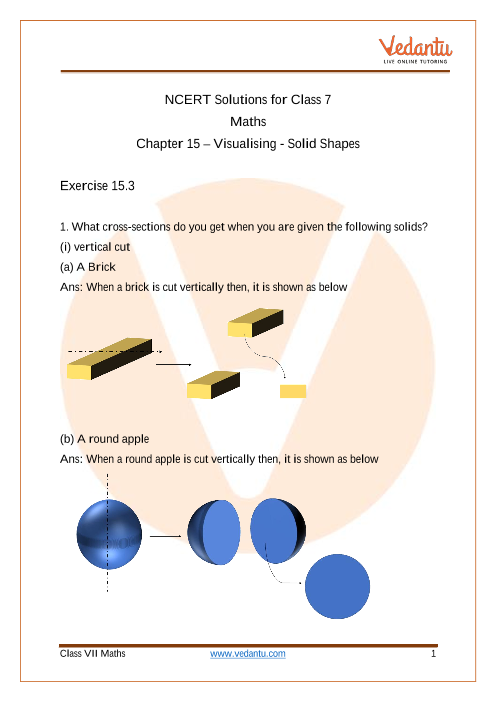Book your Free Demo session
Get a flavour of LIVE classes here at VedantuVedantu Improvement Promise
We promise improvement in marks or get your fees back. T&C Apply*

## Access NCERT Solutions for Class 7 Chapter 15 – Visualising - Solid Shapes

Exercise 15.3

1. What cross-sections do you get when you are given the following solids?

(i) vertical cut

(a) A Brick

Ans: When a brick is cut vertically then, it is shown as below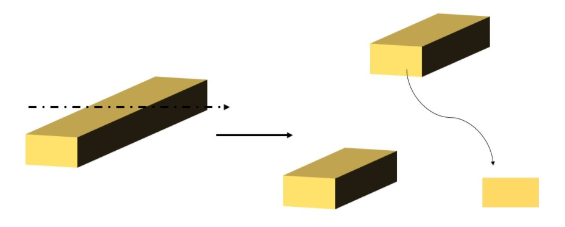(b) A round apple

Ans: When a round apple is cut vertically then, it is shown as below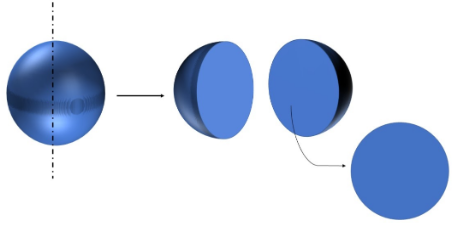(c) A die

Ans: When a die is cut vertically then, it is shown as below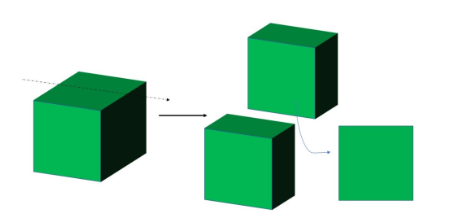(d) A particular pipe

Ans: When a particular pipe is cut vertically then, it is shown as below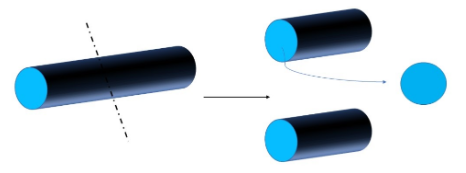(e) An ice cream cone

Ans: When an ice cream cone is cut vertically then, it is shown as below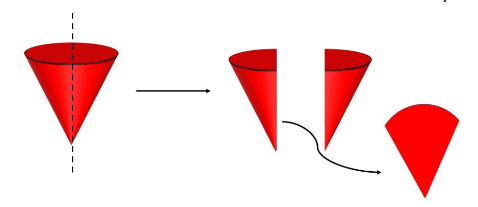(ii) Horizontal cut

(a) A brick

Ans: When a brick cone is cut horizontally then, it is shown as below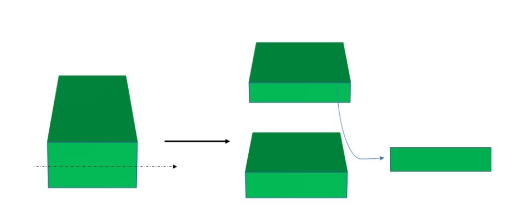(b) A round apple

Ans: When a round apple cone is cut horizontally then, it is shown as below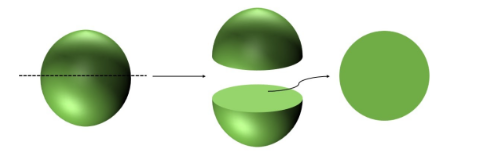(c) A die

Ans: When a die is cut horizontally then, it is shown as below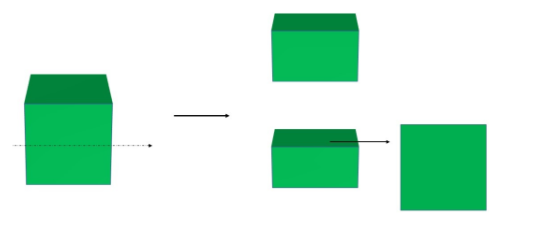(d) A particular pipe

Ans: When a particular pipe is cut horizontally then, it is shown as below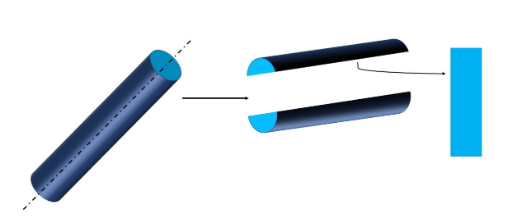(e) An ice cream cone

Ans: When an ice cream cone is cut horizontally then, it is shown as below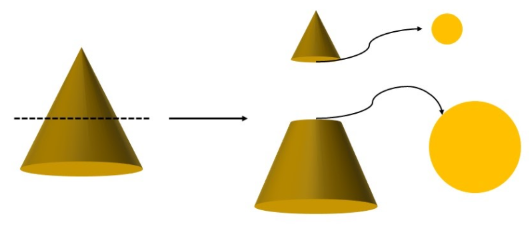## NCERT Solutions for Class 7 Maths Chapter 15 Visualising Solid Shapes Exercise 15.3

Opting for the NCERT solutions for Ex 15.3 Class 7 Maths is considered as the best option for the CBSE students when it comes to exam preparation. This chapter consists of many exercises. Out of which we have provided the Exercise 15.3 Class 7 Maths NCERT solutions on this page in PDF format. You can download this solution as per your convenience or you can study it directly from our website/ app online.

Vedantu in-house subject matter experts have solved the problems/ questions from the exercise with the utmost care and by following all the guidelines by CBSE. Class 7 students who are thorough with all the concepts from the Subject Visualising Solid Shapes textbook and quite well-versed with all the problems from the exercises given in it, then any student can easily score the highest possible marks in the final exam. With the help of this Class 7 Maths Chapter 15 Exercise 15.3 solutions, students can easily understand the pattern of questions that can be asked in the exam from this chapter and also learn the marks weightage of the chapter. So that they can prepare themselves accordingly for the final exam.

Besides these NCERT solutions for Class 7 Maths Chapter 15 Exercise 15.3, there are plenty of exercises in this chapter which contain innumerable questions as well. All these questions are solved/answered by our in-house subject experts as mentioned earlier. Hence all of these are bound to be of superior quality and anyone can refer to these during the time of exam preparation. In order to score the best possible marks in the class, it is really important to understand all the concepts of the textbooks and solve the problems from the exercises given next to it.

Do not delay any more. Download the NCERT solutions for Class 7 Maths Chapter 15 Exercise 15.3 from Vedantu website now for better exam preparation. If you have the Vedantu app in your phone, you can download the same through the app as well. The best part of these solutions is these can be accessed both online and offline as well.

1. How do you visualize a solid shape according to Chapter 15 of Class 7 Maths?

To visualise solid shapes you have to see the hidden parts of the solid object. This can be done using different methods like cutting or slicing, shadow play, and looking at it from a third angle. These methods are explained intensively in the NCERT Solutions for Chapter 15 of Class 7 Maths that are provided by Vedantu. You can also refer to the solutions in case of doubts while solving the questions or for self-assessment.

2. What is visualising solid shapes according to Chapter 15 of Class 7?

The skill to see the hidden parts of a solid figure is defined as visualising solid shapes. The objects need to be three-dimensional with vertices, corners, edges, and faces. There are various methods used to visualise solid figures as explained in the NCERT Solutions for Chapter 15 of Class 7 Maths. The NCERT Solutions for Chapter 15 of Class 7 Maths provided by Vedantu are prepared by subject matter experts and are written in an easy to understand language to help students score high marks.

3. How many views does a solid have according to Chapter 15 of Class 7 Maths?

A solid has three views: front, side, and top view. These can be seen when looking at the solid figure from a different angle. The three views work in providing a lot of information on the solid object that is required to solve the questions covered in the chapter. You can refer to the revision notes and important questions offered by Vedantu to prepare this particular chapter for the examinations.

4. What is Euler’s Formula in Chapter 15 Visualising Solid Shapes of Class 7 Maths?

Euler’s Formula is ‘F - E + V = 2’. Here, F denotes the number of faces, E denotes the edges, and V denotes the vertices for the solid shape. This formula is for polyhedron figures, that is shapes that have their faces as a polygon. You can learn the application of this formula to solve the questions in the chapter itself. The  students should follow the theory to grasp the concept clearly.

5. How are the NCERT Solutions for Chapter 15 of Class 7 Maths helpful?

Chapter 15 Visualising  Solid Shapes of Class 7 Maths can be a little complicated for the students to understand. It is necessary to comprehend the theory to solve the questions. The NCERT Solutions for this chapter have all the concepts in a simplified manner for the students. It helps the students grasp the topics and prepare well for the exams. You can refer to the NCERT Solutions for Chapter 15 of Class 7 Maths provided at free of cost on the Vedantu website and on the Vedantu app to understand the concepts better.SHARETWEETSHARESUBSCRIBE